Download Presentation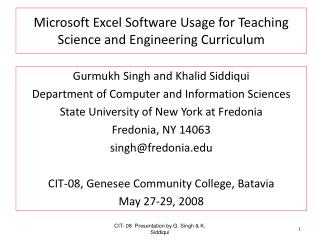Microsoft Excel Software Usage for Teaching Science and Engineering Curriculum

# Microsoft Excel Software Usage for Teaching Science and Engineering Curriculum - PowerPoint PPT Presentation

Microsoft Excel Software Usage for Teaching Science and Engineering Curriculum Gurmukh Singh and Khalid Siddiqui Department of Computer and Information Sciences State University of New York at Fredonia Fredonia, NY 14063 singh@fredonia.edu CIT-08, Genesee Community College, BataviaI am the owner, or an agent authorized to act on behalf of the owner, of the copyrighted work described.
Download Presentation## Microsoft Excel Software Usage for Teaching Science and Engineering Curriculum

An Image/Link below is provided (as is) to download presentation

Download Policy: Content on the Website is provided to you AS IS for your information and personal use and may not be sold / licensed / shared on other websites without getting consent from its author.While downloading, if for some reason you are not able to download a presentation, the publisher may have deleted the file from their server.

- - - - - - - - - - - - - - - - - - - - - - - - - - E N D - - - - - - - - - - - - - - - - - - - - - - - - - -
Presentation Transcript### Microsoft Excel Software Usage for Teaching Science and Engineering Curriculum

Gurmukh Singh and Khalid Siddiqui

Department of Computer and Information Sciences

State University of New York at Fredonia

Fredonia, NY 14063

singh@fredonia.edu

CIT-08, Genesee Community College, Batavia

May 27-29, 2008

CIT- 08 Presentation by G. Singh & K. Siddiqui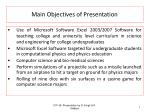### Main Objectives of Presentation

Use of Microsoft Software Excel 2003/2007 Software for teaching college and university level curriculum in science and engineering for college undergraduates

Microsoft Excel Software targeted for undergraduate students in computational physics and physics education

Computer science and bio-medical sciences

Perform simulations of a projectile such as a missile launched from an airplane to hit a target on ground for physics majors

Rolling of nine dice with six surfaces in a casino game for computer science majors

CIT- 08 Presentation by G. Singh & K. SiddiquiDevelopment and advancement in high speed micro-computers such as IBM and Mac based PCs

• Portable laptops as versatile class-room tools to teach undergraduate science and engineering curriculum
• Microcomputer machines employ several software systems such as Excel, Access, Word, PowerPoint, Groove, InfoPath, OneNote, Outlook, Publisher, FrontPage etc.
• Object oriented computing languages like C++, C#, Visual Basic (VB), Java Script, SQL etc.
• Such software systems are extensively used for undergraduate, graduates teaching in colleges, scientific labs, private companies, businesses and banks in world

### Why Microsoft Excel in College?

CIT- 08 Presentation by G. Singh & K. SiddiquiWhy Microsoft Excel 2007 in College?

• Adoption of internet technologies in undergraduate science and engineering curricula
• International/National conferences to enhance and share the knowledge gathered with other educators and researchers
• Use of Internet technologies to interactively teach in undergraduate and graduate classroom setting or during distant learning in virtual universities, which is a very effective teaching tool for the science and engineering curricula

CIT- 08 Presentation by G. Singh & K. Siddiqui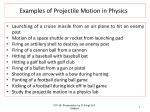Examples of Projectile Motion in Physics

• Launching of a cruise missile from an air plane to hit an enemy post
• Motion of a space shuttle or rocket from launching pad
• Firing an artillery shell to destroy an enemy post
• Firing of a cannon ball from a cannon
• Hitting of a baseball with baseball bat
• Hitting a golf ball with golf club
• Firing of a bullet from a gun or pistol
• Shooting of an arrow with a bow during hunting
• Punting of a football during ball game
• Kicking of a football during kick off in ball game
• Study the projectile motion in a physics lab

CIT- 08 Presentation by G. Singh & K. Siddiqui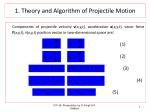Components of projectile velocity v(x,y,t), acceleration a(x,y,t), vecor force F(x,y,t), r(x,y,t) position vector in two-dimensional space are:

• (1)
• (2)
• (3)
• (4)
• (5)

### 1. Theory and Algorithm of Projectile Motion

CIT- 08 Presentation by G. Singh & K. Siddiqui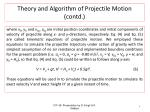where x0, y0 and v0x, v0y are initial position coordinates and initial components of velocity of projectile along x- and y-directions, respectively. Eq. (4) and Eq. (5) are called kinematic equations of projectile motion. We employed these equations to simulate the projectile trajectory under action of gravity with the simplest assumption of no air resistance and implemented boundary conditions for the present problem (i.e. ax = 0, ay = g = -9.80 m/s2, vy = V,v0y = V0,y = H, and y0 = H0), so that Eq. (4) and Eq. (5) could be written as follows along y-axis

V = Vo+ gt, H’ = Ho + Vot + 0.5gt2. (6)

These equations will be used in to simulate the projectile motion to simulate its exact velocity V and exact height H’ at a given instant of time.

### Theory and Algorithm of Projectile Motion (contd.)

CIT- 08 Presentation by G. Singh & K. Siddiqui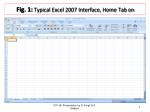Fig. 1: Typical Excel 2007 Interface, Home Tab on

CIT- 08 Presentation by G. Singh & K. Siddiqui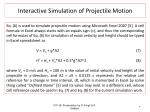Interactive Simulation of Projectile Motion

Eq. (6) is used to simulate projectile motion using Microsoft Excel 2007 . A cell formula in Excel always starts with an equals sign (=), and thus the corresponding cell formulas of Eq. (6) for simulation of exact velocity and height should be typed in Excel spreadsheet as

V = Vo+ g*A2 (7)

H’ = Ho + Vo*A2 + 0.5*g*A2^2 (8)

where Vo = 0 m/s and Ho = 100 m is the value of initial velocity and height of the projectile in y-direction, and A2 = dt = 0.0125 s represents the relative cell reference for a change in time interval, dt, which is memorized in Excel by some thing called “Defined Name”  and its value may exist in a different cell, whose cell reference could be used in Eq. (7) and Eq. (8) for the current simulation work.

CIT- 08 Presentation by G. Singh & K. Siddiqui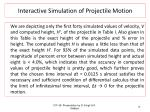Interactive Simulation of Projectile Motion

We are depicting only the first forty simulated values of velocity, V and computed height, H’, of the projectile in Table I. Also given in this Table is the exact height of the projectile and % error in height. The computed height H is always a little less than that of the exact height H’. For 93% of the simulated data points, the magnitude of percent error between simulated height and actual eight is < 4.0%, which indicates that the accuracy in computed values of projectile height is pretty good, which further proves that the chosen time interval dt = 0.0125 s almost satisfies the necessary and sufficient condition of differential calculus that in the limit of infinitesimal time interval, Δt → 0 for the projectile motion.

CIT- 08 Presentation by G. Singh & K. SiddiquiTable 1:Partial Results of Interactive Simulation

CIT- 08 Presentation by G. Singh & K. SiddiquiHorizontal Range of Projectile Motion

For 99% of the simulated values, magnitude of percent error between computed height and actual height is < 2.0%, which indicates that the accuracy in computed values of projectile height is pretty good.

The magnitude of horizontal range, R, of projectile during its time of flight t = 4.775, assuming a constant speed of airplane, Vairplane = 500 miles/hour along x-axis, can be obtained from kinematic equation Eq. (4) by using the initial boundary conditions, i.e., x = R, ax = 0, x0 = 0 and v0x = Vairplane:

R = tVairplane = 1067 m (9)

R is the distance where the projectile will hit a target on the ground. In the present problem, R = 1.07 km, which can be increased either by increasing airplane’s speed with respect to ground or by imparting some initial thrust to the projectile at launch time or by a combination of both.

CIT- 08 Presentation by G. Singh & K. SiddiquiFig. 2: A plot of projectile height, H versus time, t

CIT- 08 Presentation by G. Singh & K. Siddiqui1

2

Two slider bars are used to perform simulations with different initial velocity V0 of the projectile and at a different initial height H0 of the airplane. Slide bar 1 represents the instantaneous initial height of the projectile, whereas slide bar 2 shows the initial velocity of the projectile at launch time. The initial height, H0 and initial velocity, V0 of the projectile can be increased or decreased by clicking on right or left hand side arrow existing on each end of a slide bar.

CIT- 08 Presentation by G. Singh & K. Siddiqui2. Interactive Simulations of Nine Rolling Dice

To simulate rolling of nine dice in a casino game, we employ latest version of Microsoft Excel 2007 and use a built-in pseudo number generating function, RAND( ), which can generate fractional numbers between 0 and 1. As none of the faces of a dice has marked with zero a dot, one is should include this fact while generating the random numbers. Cell formula to create non-zero random numbers for the rolling of nine dice should also include a factor of 6, which is multiplied by the function RAND( ) to take into account the fact of six faces of a dice, and a factor of unity is added to it to exclude zero value generated random numbers. The random numbers thus generated for nine rolling dice are given in Table 2 in its first nine columns.

CIT- 08 Presentation by G. Singh & K. SiddiquiInteractive Simulations of Nine Rolling Dice

The random numbers thus generated for nine rolling dice are given in Table 2 in its first nine columns. Column ten shows the sum total of scores obtained for all the nine dice in one trial. Eleventh column represents the ratio of sum total score of all nine dice in one row to the maximum score among all 200 data values in column ten of Table 2. If one double clicks any cell of generated data, and then hits the ENTER key on the keyboard, all simulated random numbers for nine dice will change instantaneously and consequently, the total score in a single row normalized with the maximum score of the tenth column data values will also change.

CIT- 08 Presentation by G. Singh & K. Siddiqui### Table 2:Simulated value of number of dots on the six faces of each dice in rolling of nine dice

CIT- 08 Presentation by G. Singh & K. Siddiqui### Fig. 4: A plot of ratio of total score in one row to the maximum score as a function of number of trials

CIT- 08 Presentation by G. Singh & K. SiddiquiInteractive Simulations of Nine Rolling Dice

In Fig. 4, we display a graph of this normalized total score as a function of number of trials. This graph has several peaks and valleys and it looks like the replica of an Electrocardiograph (ECG), which is obtained for a patient with some defect in the heart causing an irregular heart-beat. The interactive plot of Fig. 4 has in general, one or two peaks with a maximum value equals unity, and the remaining peaks always have values less than unity. The location of the maximum peak values and the nature of the plot changes with each new simulation, showing pretty interesting application of Excel 2007 for computer science and medical undergraduates.

CIT- 08 Presentation by G. Singh & K. SiddiquiIn conclusion, we may emphasize that the present paper has quite important implications both in physics as well as in computer science and medical science curriculum:

• In physics the students will learn how to employ software system such as Excel 2007 [1, 5] to simulate the basic concept of projectile motion under the action of constant gravitational acceleration with no air resistance
• Whereas in computer science, they could visualize the real time application of this fundamental concept of physics in a virtual laboratory.
• In addition, medical students can have an idea of irregular heart-beat of a patient suffering from heart attack or stroke, which has been proven with the help of a plot of normalized total score as a function of the number of trials from the simulations of nine rolling dice.

### Concluding Remarks

CIT- 08 Presentation by G. Singh & K. Siddiqui